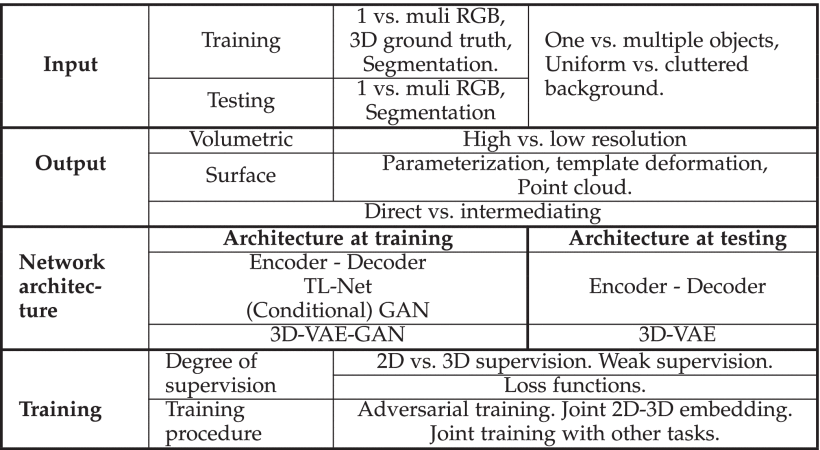# (작성중)[survey 논문 정리] Image-based 3D object Reconstruction: State-of-the-Art and Trends in the Deep Learning Era

leehyuna·2022년 3월 31일
2

## 논문 리뷰

목록 보기
2/3

Image-based 3D object Reconstruction: State-of-the-Art and Trends in the Deep Learning Era

### 2. Problem statement and Taxonomy

• 본 paper에서 사용되는 용어, 수식 정리

• $I = \{ I_k,k=1,..., n \}, \ n ≥ 1$
: 하나 또는 그 이상의 객체 X 의 RGB 이미지
• $f_θ$
: shape $X'$을 추론하는 predictor
: reconstruction objective $L(I)=d(f_θ(I), X)$를 최소화 하는 함수
• $θ$
: a set of parameter of $f$
• $d(•,•)$
: target shape $X$와 reconstructed shape $f(I)$ 의 차이를 측정하는 함수
• $L$
: reconstruction objective == loss funtion in the deep learning literature

이 서베이는 최신 방법들을 아래 다섯 카테고리로 나누고 설명한다.

1. the nature of input I (입력 I의 성질)
2. the representation of the output (출력 표현)
3. the deep learning network architecture used during training and testing to approximate the predictor f (학습과 평가에 사용하는 딥러닝 네트워크 구조)
4. the training procedure (학습 과정)
5. the degree of supervision• 1. Input
• a single image
• multiple images
• captured using RGB cameras whose intrinsic and extrinsic parameters can be known or unknown
• a video stream
• i.e., a sequence of images with temporal correlation
• 2. Representation of the output
- 출력 표현은 네트워크 구조를 선택하는 데에 중요하다.
또한, 계산 효율성과 reconstruction quality에 영향을 미친다.

• Volumetric representations
• 초기 딥러닝 기반 3D reconstruction 기술에 채택되어 널리 사용되었다.
• regular voxel grids를 사용하는 표현 방식이다.
• 이미지 분석에 사용되는 2D convolutions은 3D로 쉽게 확장될 수 있다.
• 하지만, memory 사용량이 많고, 적은 기술만이 sub-voxel accuracy를 달성했다.
• Surface-based representations
• meshes, point clouds 표현이 여기에 속한다.
• 이 표현 방식은 메모리 효율적이지만, 이러한 표현은 규칙적인 구조가 아니어서 딥러닝 구조에 잘 들어맞지 않는다.
• Intermediation
• 몇몇 3D reconstruction 알고리즘은 RGB이미지로부터 바로 물체의 3D geometry를 예측한다.
• 다른 몇몇은 순차적인 단계로 문제를 나누어 해결한다. 각 단계는 중간 표현을 예측한다.
• 3. Network architecture

Predictor $f$를 구현하기 위해 다양한 network 구조가 사용되었다. Backbone 구조는 encoder $h$와 그 뒤를 따르는 decoder $g$로 구성된다.

$f = h•g$